Trigonometry Formulas for class 11 (PDF download)

Trigonometry is quite a interesting subject. Here are the useful Trigonometry Formulas for class 11 Maths

Basic Formula

$tan(x) = \frac {sin(x)}{cos(x)}$

$cot(x) = \frac {cos(x)}{sin(x)}$

Reciprocal Identities:

$cosec(x) = \frac {1}{sin(x)}$

$sec(x) =\frac { 1}{cos(x)}$

$cot(x) = \frac {1}{tan(x)}$

$sin(x) = \frac {1}{cosec(x)}$

$cos(x) = \frac {1}{sec(x)}$

$tan(x) = \frac {1}{cot(x)}$

Pythagorean Identities:

$sin^2(x) + cos^2(x) = 1$

$cot^2x +1 = cosec^2x$

$1+tan^2x = sec^2x$

Trigonometric Ratio’s of Common angles

We can find the values of trigonometric ratio’s various angle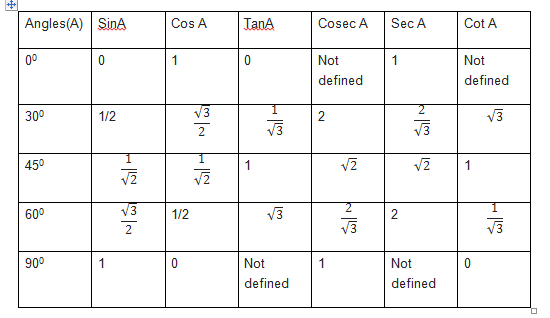Trigonometry Formula for Complementary and supplementary angles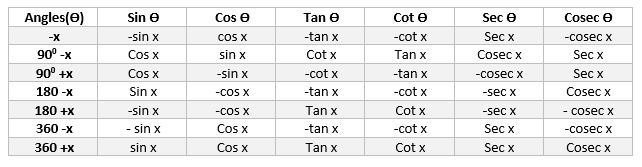Sin and cos function

1. $cos(A+B)=cos(A)cos(B)-sin(A)sin(B)$
2. $cos(A-B)=cos(A)cos(B)+sin(A)sin(B)$
3. $cos(\pi /2 -A)=sin(A)$
4. $sin(\pi /2 -A)=cos(A)$
5. $sin(A+B)=sin(A)cos(B)+sin(B)cos(A)$
6. $sin(A-B)=sin(A)cos(B)-sin(B)cos(A)$

Tan and cot functions

If none of the angles x, y and (x + y) is an odd multiple of $\pi /2$

$tan(A+B)=\frac{tan(A)+tan(B)}{1-tan(A)tan(B)}$

$tan(A-B)=\frac{tan(A)-tan(B)}{1+tan(A)tan(B)}$

If none of the angles x, y and (x + y) is an multiple of $\pi /2$

$cot(A+B)=\frac{cot(A)cot(B)-1}{cot(A)+cot(B)}$

$cot(A-B)=\frac{cot(A)cot(B)+1}{cot(B)-cot(A)}$

Some more Trigonometric Functions
Double of x
$cos2x=cos^{^{2}}x-sin^{^{2}}x=2cos^{^{2}}x-1=1-2sin^{^{2}}x=\frac{1-tan^{^{2}}x}{1+tan^{^{2}}x}$
$sin2x=2cos(x)sin(x)=\frac{2tan(x)}{1+tan^{^{2}}x}$
$tan2x=\frac{2tan(x)}{1-tan^{^{2}}x}$
Triple of x
$sin3x=3sin(x)-4sin^{3}x$
$cos3x=4cos^{3}x-3cos(x)$

$tan(3x)=\frac{3tanx-tan^{^{3}}x}{1-3tan^{^{2}}x}$

Some other Important functions

$cos(A)+cos(B)=2cos\frac{A+B}{2}cos\frac{A-B}{2}$
$cos(A)-cos(B)=-2sin\frac{A+B}{2}sin\frac{A-B}{2}$
$sin(A)+sin(B)=2sin\frac{A+B}{2}cos\frac{A-B}{2}$
$sin(A)-sin(B)=2cos\frac{A+B}{2}sin\frac{A-B}{2}$

Half Angle Formula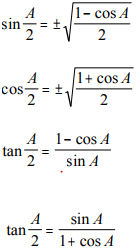Pythagoras Identities in Radical form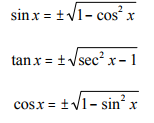Power Reducing Functions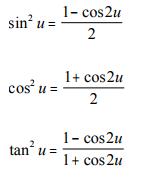Trigonometric equations Formula’s

1.$sin x = 0$ implies  $x = n \pi$, where n is any integer<br>
2.$cos x = 0$ implies $x = (2n + 1)(\pi /2)$<br>

1. $sinx =siny$ then $x=n \pi + (-1)^{n}y$ where n is any integer<br>
2. $cosx=cosy$ then $x=2n \pi + y$ or $x=2n \pi – y$ where n is any integer<br>
3. $tanx=tany$ then $x=n \pi +y$ where n is any integer<br>

5.Equation of the form
$sin^2x = sin^2 y, cos^2 x = cos^2 y , tan^2 x = tan^2 y$
General solution is given by
$x = n \pi \pm y$ where n is any integer

6.Equation of the form<br>
|sin x|=1 ,General solution is given by $x= (2n+1) \frac {\pi}{2}$<br>
|cos x|=1,General solution is given by $x=n \pi$<br>

Some basics Tips to solve the trigonometry questions

1) Always try to bring the multiple angles to single angles using basic formula. Make sure all your angles are the same. Using sin(2X) and sinX is difficult, but if you use sin2X = 2sin(x)cos(x), that leaves sin(x) and cos(x), and now all your functions match. The same goes for addition and subtraction: don’t try working with sin(X+Y) and sinX. Instead, use sin(X+Y) = sin(x)cos(y)+cos(x)sin(y) so that all the angles match

2) Converting to sin and cos all the items in the problem using basic formula. I have mentioned sin and cos as they are easy to solve.You can use any other also.

3)Check all the angles for sums and differences and use the appropriate identities to remove them.

4) Use Pythagorean identifies  to simplify the equations

5) Practice and Practice. You will soon start figuring out the equation and there symmetry to resolve them fast

Trigonometric functions

Domain ,Range and Graphs of Trigonometric functions

Trigonometric equations

Trigonometric table from 0 to 360 (cos -sin-cot-tan-sec-cosec)

Important trigonometry questions for class 11 Maths

How to easily remember trigonometric ratios table

Trigonometry-Formulas
Trigonometry-Formulas.pdf
261.0 KiB